# TLA+ 笔记

TLA+ Tools 包含很多工具，可以在这里下载，大部分人都是使用这个 Tools：

## 介绍

2016 年开始微软在内部办了一共三天的 TLA+ School，教学了很多微软工程师 TLA+，一共运行了三次，到第四次时，Lamport 终于受不了重复给别人讲 TLA+ 基础，于是做了教学视频，没错就是很火的这个视频：http://lamport.azurewebsites.net/video/videos.html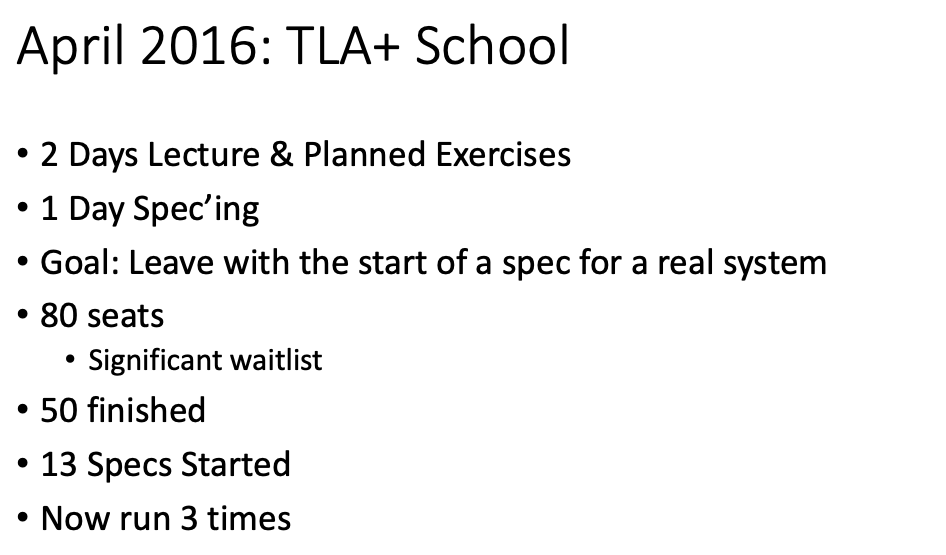## Learn TLA+ 笔记

### Introduction & PlusCal

PlusCal 的基本语法：

---- MODULE module_name ----
\* TLA+ code

(* --algorithm algorithm_name
begin
\* PlusCal code
end algorithm; *)
====


---- MODULE transfer ----
EXTENDS Naturals, TLC

(* --algorithm transfer
variables alice_account = 10, bob_account = 10, money = 5;

begin
A: alice_account := alice_account - money;
B: bob_account := bob_account + money;

end algorithm *)
====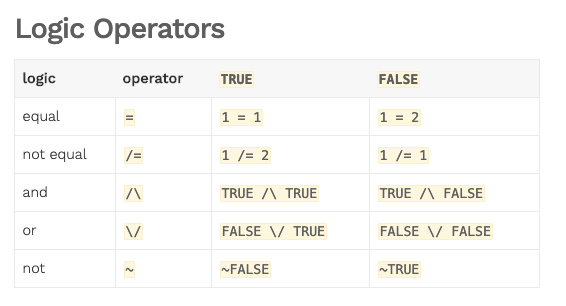---- MODULE Transfer ----
EXTENDS Naturals, TLC

(* --algorithm transfer
variables alice_account = 10, bob_account = 10,
account_total = alice_account + bob_account;

process Transfer \in 1..2
variable money \in 1..20;
begin
Transfer:
if alice_account >= money then
A: alice_account := alice_account - money;
bob_account := bob_account + money;
end if;
C: assert alice_account >= 0;
end process

end algorithm *)

MoneyNotNegative == money >= 0
MoneyInvariant == alice_account + bob_account = account_total

====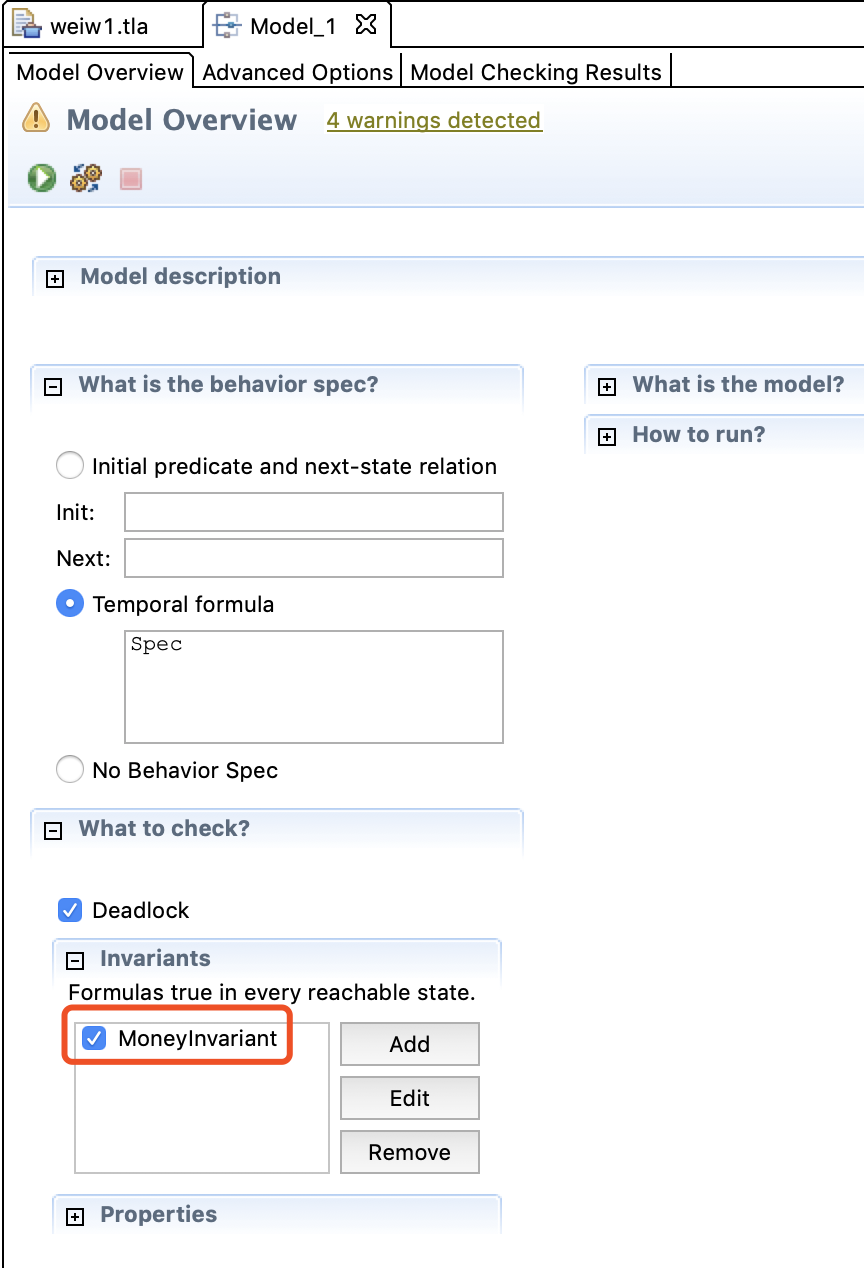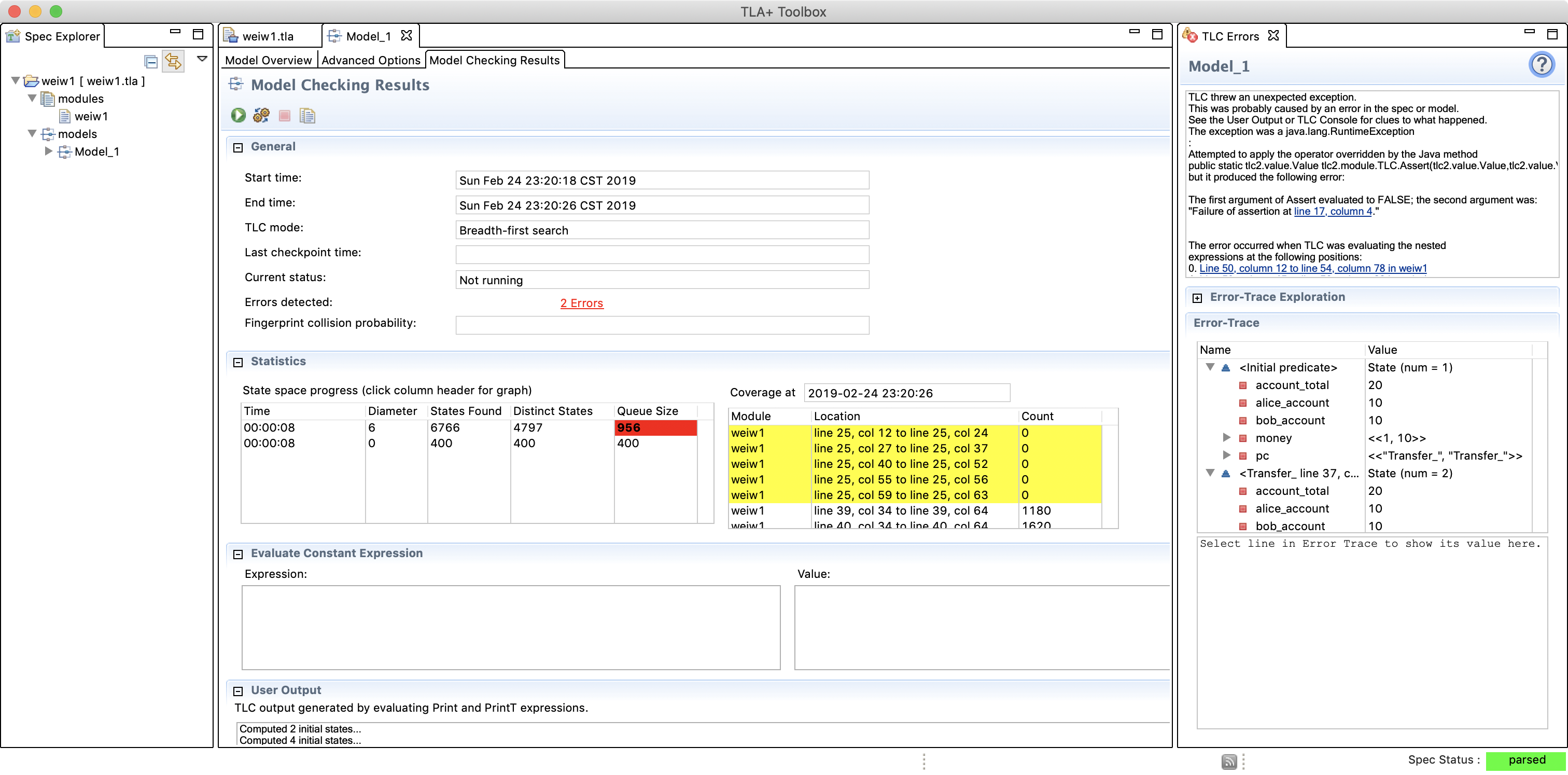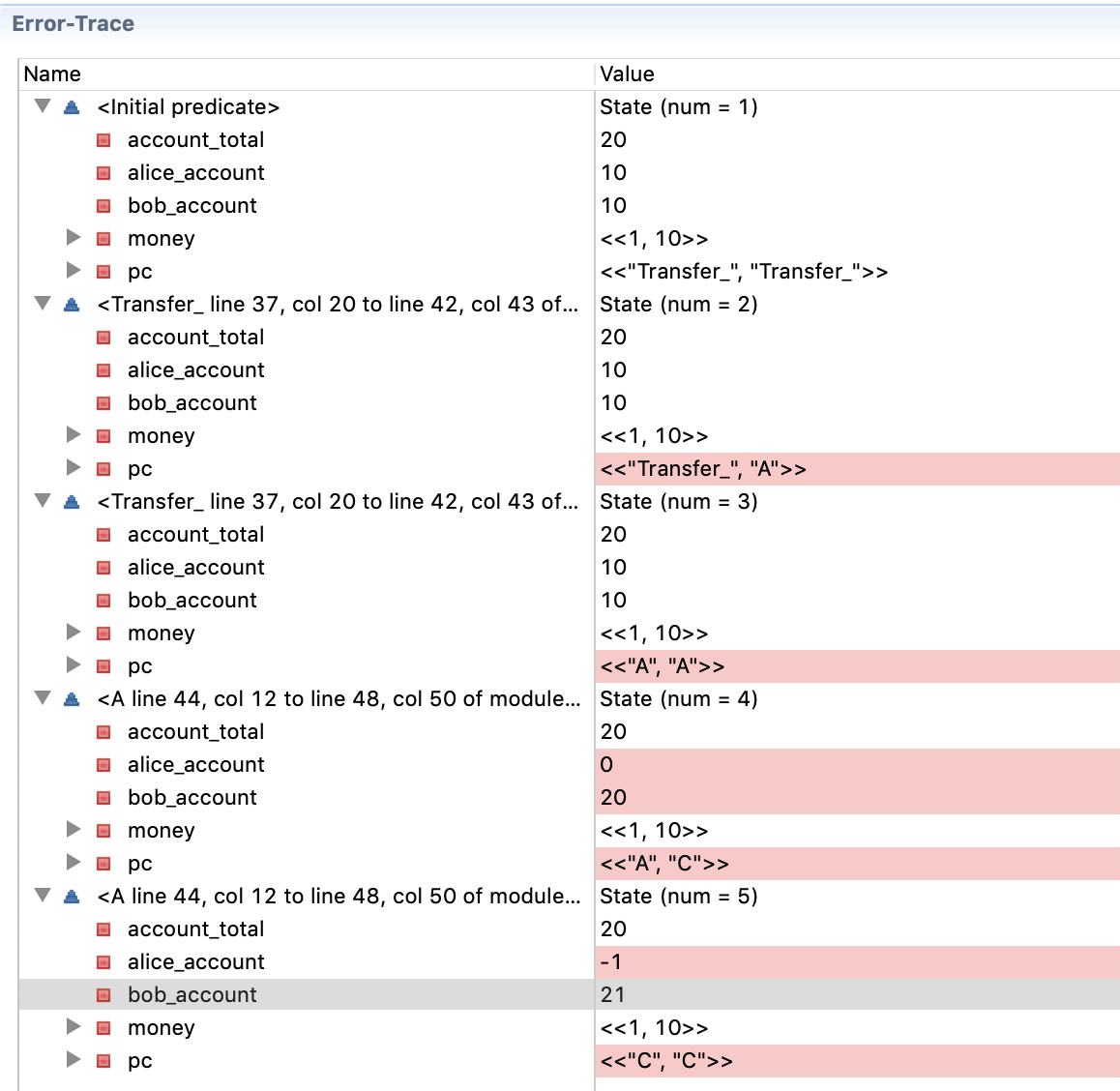PlusCal 中是有 print 的，比如下面这个例子：

EXTENDS TLC

(* --algorithm hello_world
variable s \in {"Hello", "World!"};
begin
A:
print s;
end algorithm; *)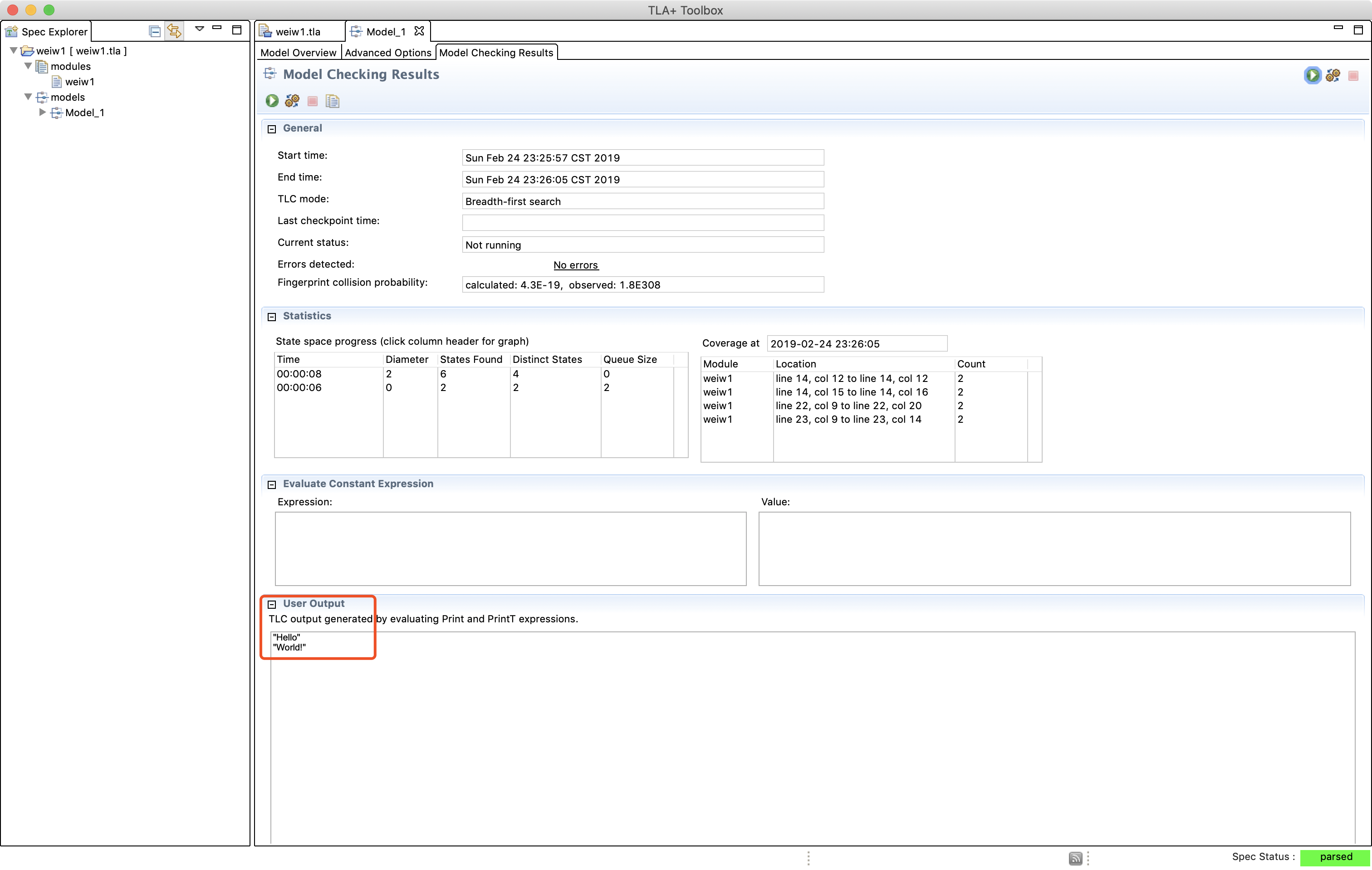PlusCal 的 if、while、goto 都和一般语言很像，就不赘述了。

Model Overview 里的 “no behavior spec” 一般很少用，运行后在 result 页可以使用 “evaluate constant expression”，然后可以写点东西来验证 TLA+ 是如何运行的。

either 简单地说就是没有条件的 if，可以让 TLC 知道这里有两种路径，都可以执行以下：

variables x = 3, i = 2;
begin
while i > 0 do
either
x := x + 2;
or
x := x * 2;
end either;
i := i - 1;
end while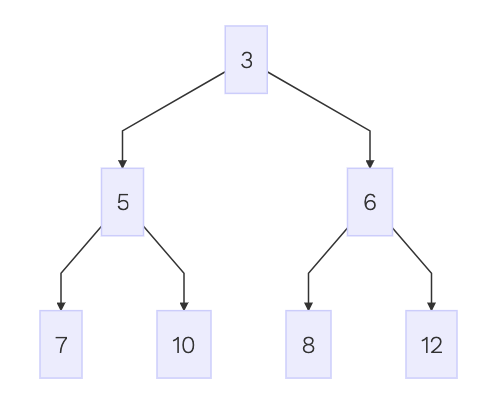with a \in {1, 2, 3} do
x := x + a
end with;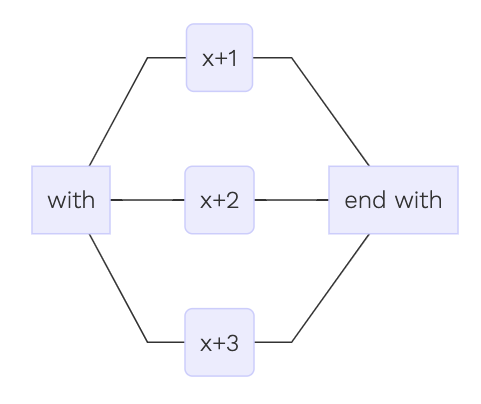Recent comment authors